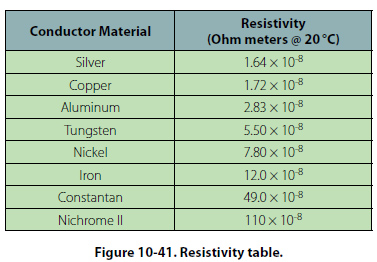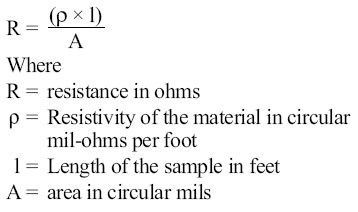Resistance of a Conductor While wire of any size or resistance value may be used, the word “conductor" usually refers to materials that offer low resistance to current flow, and the word “insulator" describes materials that offer high resistance to current. There is no distinct dividing line between conductors and insulators; under the proper conditions, all types of material conduct some current. Materials offering a resistance to current flow midway between the best conductors and the poorest conductors (insulators) are sometimes referred to as “semiconductors," and find their greatest application in the field of transistors. The best conductors are materials, chiefly metals, which possess a large number of free electrons; conversely, insulators are materials having few free electrons. The best conductors are silver, copper, gold, and aluminum; but some nonmetals, such as carbon and water, can be used as conductors. Materials such as rubber, glass, ceramics, and plastics are such poor conductors that they are usually used as insulators. The current flow in some of these materials is so low that it is usually considered zero. The unit used to measure resistance is called the ohm. The symbol for the ohm is the Greek letter omega (O). In mathematical formulas, the capital letter “R" refers to resistance. The resistance of a conductor and the voltage applied to it determine the number of amperes of current flowing through the conductor. Thus, 1 ohm of resistance will limit the current flow to 1 ampere in a conductor to which a voltage of 1 volt is applied. Factors Affecting Resistance The resistance of a metallic conductor is dependent on the type of conductor material. It has been pointed out that certain metals are commonly used as conductors because of the large number of free electrons in their outer orbits. Copper is usually considered the best available conductor material, since a copper wire of a particular diameter offers a lower resistance to current flow than an aluminum wire of the same diameter. However, aluminum is much lighter than copper, and for this reason as well as cost considerations, aluminum is often used when the weight factor is important. The resistance of a metallic conductor is directly proportional to its length. The longer the length of a given size of wire, the greater the resistance. Figure 10-40 shows two wire conductors of different lengths. If 1 volt of electrical pressure is applied across the two ends of the conductor that is 1 foot in length and the resistance to the movement of free electrons is assumed to be 1 ohm, the current flow is limited to 1 ampere. If the same size conductor is doubled in length, the same electrons set in motion by the 1 volt applied now find twice the resistance;consequently, the current flow will be reduced by one-half. The resistance of a metallic conductor is inversely proportional to the cross-sectional area. This area may be triangular or even square, but is usually circular. If the cross-sectional area of a conductor is doubled, the resistance to current flow will be reduced in half. This is true because of the increased area in which an electron can move without collision or capture by an atom. Thus, the resistance varies inversely with the cross-sectional area of a conductor. The fourth major factor influencing the resistance of a conductor is temperature. Although some substances, such as carbon, show a decrease in resistance as the ambient (surrounding) temperature increases, most materials used as conductors increase in resistance as temperature increases. The resistance of a few alloys, such as constantan and Manganin™, change very little as the temperature changes. The amount of increase in the resistance of a 1 ohm sample of a conductor, per degree rise in temperature above 0° Centigrade (C), the assumed standard, is called the temperature coefficient of resistance. For each metal, this is a different value; for example, for copper the value is approximately 0.00427 ohm. Thus, a copper wire having a resistance of 50 ohms at a temperature of 0 °C will have an increase in resistance of 50 × 0.00427, or 0.214 ohm, for each degree rise in temperature above 0 °C. The temperature coefficient of resistance must be considered where there is an appreciable change in temperature of a conductor during operation. Charts listing the temperature coefficient of resistance for different materials are available. Figure 10-41 shows a table for “resistivity" of some common electric conductors.The resistance of a material is determined by four properties: material, length, area, and temperature. The first three properties are related by the following equation at T = 20 °C (room temperature):©AvStop Online Magazine                                                                                                                                                      Contact Us              Return To Books